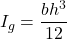# Cracking Moment

#### EngineeringFabrication & Quality

The cracking of concrete occurs due to several reasons. These reasons include excessive deflection, plastic shrinkage, severe corrosion of steel rebar, etc. However, the concept of cracking moment deals with the cracking of concrete beams due to deflection.

In this article, you will learn the definition of cracking moment, its equation, how to calculate it, and its use pertaining to beams.

## Definition

The cracking moment denotes the tensile bending stress at which concrete will start to crack. In practice, concrete excels at withstanding compressive loads but fails under tension. Thus, engineers often use steel to reinforce concrete and improve its tension capacity.

Nevertheless, because the reinforcing steel cross-sectional area is typically 2% or less of the concrete beam cross-section, the beam properties do not change significantly. As a result, they still experience cracking under excessive tensile loads.

The figures above show the relationship between loading and deflection of a concrete beam. Also, they show how cracking of the beam progresses and affects the beam’s flexural rigidity (EI). Moreover, the onset of cracking (point ‘A’) is typically from the ends of the beam, meaning that this point coincides with the cracking moment.

## Cracking Moment Equation

The cracking moment of concrete beam (Mcr) is a function of its gross moment of inertia (Ig), its modulus of rupture (fr), and the distance from its center of gravity (centroid) to the extreme fiber on the tension side of the beam (yt).## How to Calculate Cracking Moment

The cracking moment equation shows that the determination of other parameters is a prerequisite to obtaining the moment value. Hence, it is necessary to take a critical look at these parameters, namely the modulus of rupture, momenta of inertia, and distance from the centroid.

### Modulus of Rupture

Modulus of rupture refers to the amount of force and compression a beam (usually concrete) can withstand before failure. Moreover, it is a measure of the beam’s resistance to bending, and an important parameter in civil construction parts. For a given concrete beam, the modulus of rupture (fr), is a function of the compression strength of the concrete mix (f’c).### Moment of Inertia

When computing the cracking moment of concrete, convention demands the determination of the gross moment of inertia. For a rectangular cross-section, the gross moment of inertia (Ig), depends on the width (b) and height (h) of the beam.Another common shape engineers utilize in construction is a circular cross-section. The gross moment of inertia for this shape relates to its radius (r) or diameter (D).### Distance from Centroid

When a concrete beam deflects under loading, it does so about its center of gravity, or centroid. In addition, the tension along the fibers of the beam varies, with the fiber furthest away from the centroid experiencing the maximum.

As a result, cracking in the concrete commences at the fiber furthest away from the centroid. Thus, its use in the cracking moment formula. For a rectangular cross-section, this distance from the centroid (yt) depends on the height (h).The accurate determination of these three parameters (modulus of rupture, moment of inertia, and distance from centroid) is vital in calculating the cracking moment of concrete.

## Use of Cracking Moment in Beams

Determining the cracking moment value for concrete serves as a basis for beam design as well as other load-bearing components.